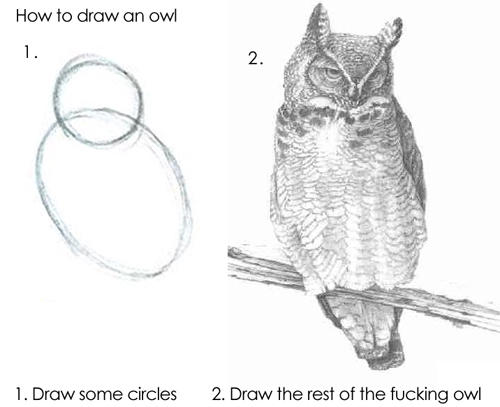# The Simplest Deep Learning Program That Could Possibly Work

Mon 15 October 2018

tags: ML

Once upon a time, when I, a C programmer, first learned Smalltalk, I remember lamenting to J.D. Hildebrand "I just don't get it: where's the `main()`?" Eventually I figured it out, but the lesson remained: Sometimes when learning a new paradigm, what you need isn't a huge tutorial, it's the simplest thing possible.

With that in mind, here is the simplest Keras neural net that does something "hard" (learning and solving XOR) :

``````import numpy as np
from keras.models import Sequential
from keras.layers.core import Activation, Dense
from keras.optimizers import SGD

# Allocate the input and output arrays
X = np.zeros((4, 2), dtype='uint8')
y = np.zeros(4, dtype='uint8')

# Training data X[i] -> Y[i]
X = [0, 0]
y = 0
X = [0, 1]
y = 1
X = [1, 0]
y = 1
X = [1, 1]
y = 0

# Create a 2 (inputs) : 2 (middle) : 1 (output) model, with sigmoid activation
model = Sequential()

# Train using stochastic gradient descent
sgd = SGD(lr=0.1, decay=1e-6, momentum=0.9, nesterov=True)
model.compile(loss='mean_squared_error', optimizer=sgd)

# Run through the data `epochs` times
history = model.fit(X, y, epochs=10000, batch_size=4, verbose=0)

# Test the result (uses same X as used for training)
print (model.predict(X))
``````

If you run this, there will be a startup time of several seconds while the libraries load and the model is built, and then you will start to see output from the call to `fit`. After the data has been run through 10,000 times, the model will then try to predict the output. As you'll see, the neural network has learned the proper set of weights to solve the XOR logic gate.

Now draw the rest of the owl.